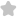C + 2 x + sec? x, then k? +1 (d) k ...
Question# C + 2 x + sec? x, then k? +1 (d) k If f(x) = cos x + Sep 2 (a) f(x) <1 15. Which of the f(x) <1 (b) f(x)=1 h of the following is incorrect? (c) 2 JEE/Engineering Exams Maths SolutionShare2174.0 (1 ratings)( f(x)=cos ^{2} x+sec ^{2} x ) ( cos ^{2} x=1-sin ^{2} x ) ( sec ^{2} x=1+tan ^{2} x ) ( f(x)=1-sin ^{2} x+tan ^{2} x+1 ) ( Rightarrow 2+frac{sin ^{2} x}{cos ^{2} x}-sin ^{2} x ) ( Rightarrow 2+frac{sin ^{2} x-sin ^{2} x cos ^{2} x}{cos ^{2} x} ) ( Rightarrow 2+frac{sin ^{2} xleft(1-cos ^{2} xright)}{cos ^{2} x} ) ( Rightarrow 2+frac{sin ^{4} x}{cos ^{2} x} ) Here ( frac{sin ^{4} x}{cos ^{2} x} geq 0 ) ( therefore f(x) geq 2 ) Hence (D) is the correct answer.2174.0 (1 ratings) Rate SolutionShareQuick and Stepwise Solutions Just click and SendOVER 20 LAKH QUESTIONS ANSWERED Download App for Free